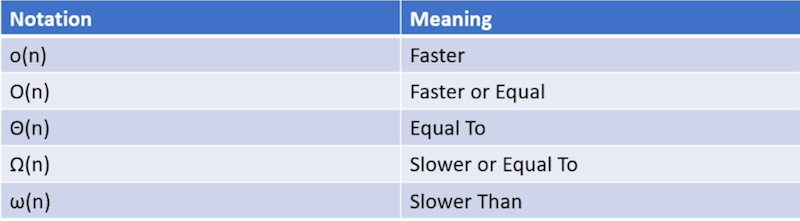# Big O Notation

03.07.2018

Episode #3 of the course Kurt Anderson by Computer science fundamentals

Welcome back!

Now that we have a good understanding of n-notation, let’s take a closer look at how we can use it to strategically compare algorithms. For this, we need to add a little bit to the n-notation. We need to add what is called a “Big O” notation to it (O(logn), O(n), etc.).

What Is Big O Notation?

Big O is actually the uppercase greek symbol, Omicron (Ө). It’s used in computer science to “set an upper bound” for run time. All this means is that it is used to tell us what the algorithm will run at its worst.

For example, take an algorithm: algorithm 1, which has a run time of O(n). This simply means that algorithm 1 will, at its worst, run n. It could run faster, but it will never be worse than n. This additional notation is important because algorithms may run differently depending on the circumstances. Let’s say we have a sorting algorithm. This algorithm might run really fast if the data is almost entirely sorted, and may run slow if it’s not.

Now, O is not the only notation we can add to the n-notation. We can distinguish anything we want with similar notations (see the table below).Why Is Big O More Commonly Used?

As computer scientists, we tend to not like surprises. Because of this, we want to use a notation that will guarantee us the worst possible case. Big O does the best job of this. It shows us just how bad an algorithm could get, so we can plan around it or possibly use an algorithm that will do better. Let’s use numbers to drive this point home a little more.

Let’s say we have a time scale to determine how fast our algorithm runs. In this scale, the closer to 0 we go, the faster our algorithm. Let’s see why Big O is typically more useful than other similar notations.

ω(7) – The algorithm will run slower, or > 7. How much greater? 1,000. 1,000,000? There is no way to know. This algorithm could take years to complete the simplest of tasks.

Ω(7) – The algorithm will run “slower or equal” to 7, or >=7. Still could run to infinity or really large run times.

Θ(7) – The algorithm is equal to 7, or = 7. This would be great. If we can guarantee that an algorithm will always run equal to something, we would use this. However, this is highly unlikely in the real world, so it’s not used too often.

O(7) – The algorithm will run “faster or equal” to 7, or <=7. This is good. We have a limit on the algorithm. We know in all cases, 7 is the worst this algorithm can do. This can be used to plan with. No surprises will come up here.

o(7) – The algorithm will run faster than 7, or < 7. There is nothing inherently wrong with this, except that it’s just less accurate than O(7). How much faster will it run? 6? 2? We don’t know the limit. Although we can still plan a worst case scenario with this, it’s less accurate than O(7), which is why it’s rarely used.

There you have it, Big O notation. This is used all across computer science, and now you know how to read it!

Key takeaways:

• Big O notation sets an upper limit to the run time of an algorithm.

• Big O is represented using an uppercase Omicron: O(n), O(nlogn), etc.

• There are other notations, but they are not as useful as O for most situations. So, O(n) is what can be seen most often.

Tomorrow, we will look at our first data structure, arrays!

Cheers,

Kurt

Recommended resources

Big O Cheat Sheet: Notice how on specific operations—for example, insert—Θ is used. This is because we can be exact. I will use O throughout the course to be consistent.

Analysis of Algorithms | Big O Analysis: Learn more about the formal math behind Big O analysis.

Share with friends